# The Coordinate System

 Our Algebra 1 tutorial software program contains over 60 topic areas. One of them is The Coordinate System, and this page summarizes the main ideas of this topic. This page is intended for review, and is not a substitute for the interactive, self-paced tutorials of the MathTutor algebra 1 software program.

General Explanation

In our study of algebra, we will often work with lines and graphs. To prepare for this, let's discuss the number line and the coordinate system.

The number line can be used to represent positive and negative numbers:
```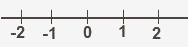```
Now try to imagine a two-dimensional number line:
```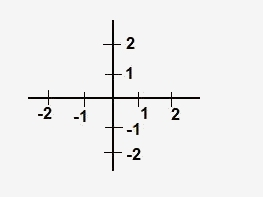```
Next, we'll label the horizontal line with the letter x and the vertical line
with the letter y.
```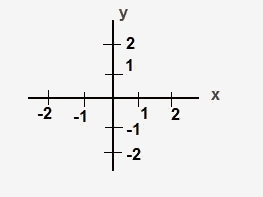```
This is called the COORDINATE SYSTEM. The horizontal line in our coordinate system is called the X-AXIS and the vertical line is called the Y-AXIS. The point where the two lines meet is called the ORIGIN.

Every point in the coordinate system can be defined by its location relative to the origin. To do this, we measure from the origin how far we traveled along the x-axis and the y-axis to reach the point. For example, the point A shown below has an x-coordinate of 2 and a y-coordinate of 1.
```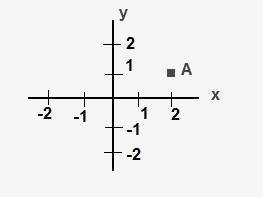```
We can write point A's coordinates as (2,1). The x-coordinate is shown first and the y-coordinate second.

Sample Problem

What are the coordinates of A in the image below?
```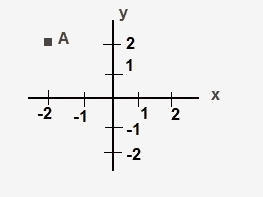```

Solution

To find the coordinates of A, do the following steps:
1. Start at (0,0).
2. Move left 2.
3. Move up 2.
4. The coordinates of A are (-2,2).